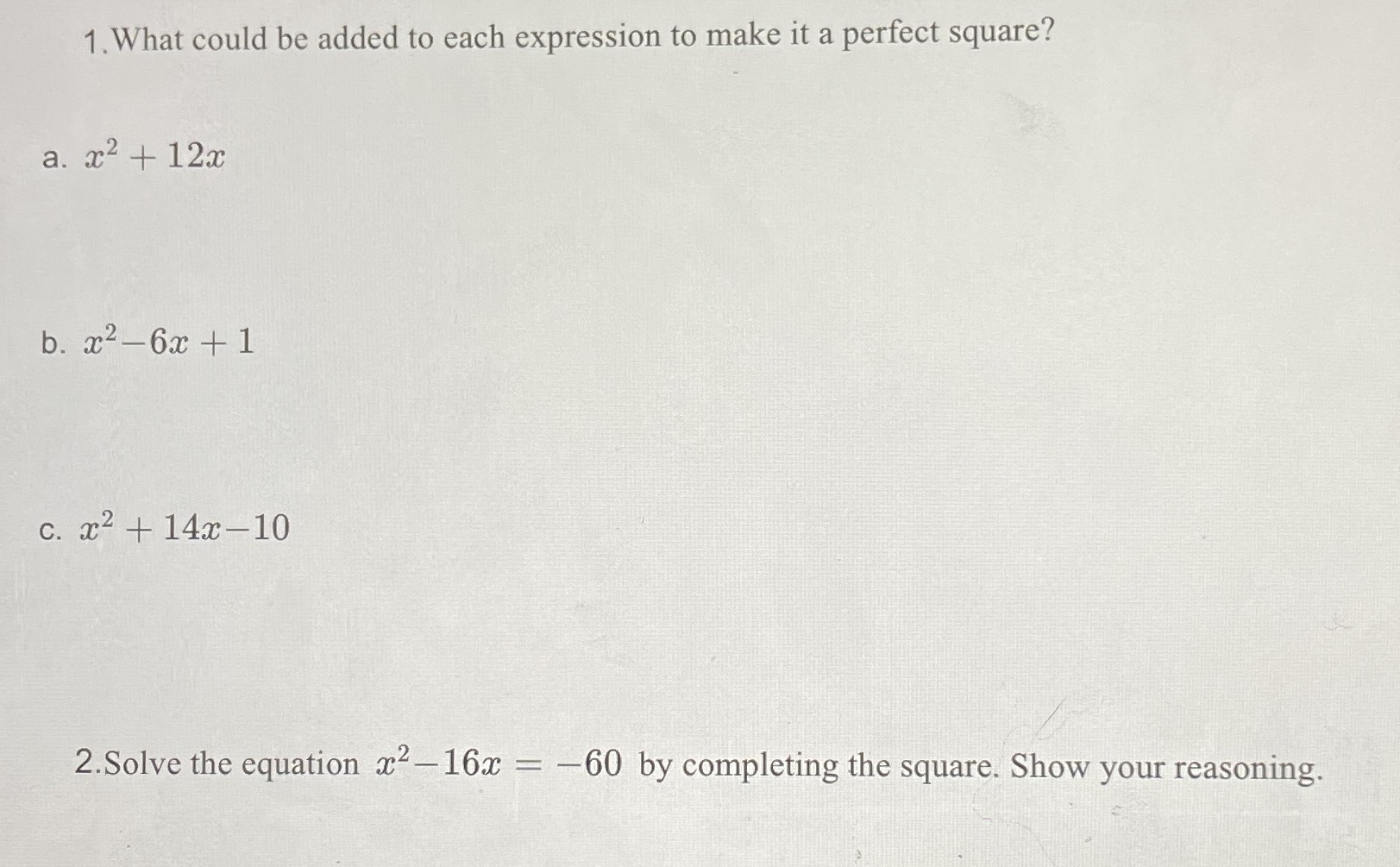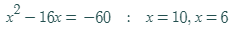### ¿Todavía tienes preguntas de matemáticas?

Pregunte a nuestros tutores expertos
Algebra
Pregunta1. What could be added to each expression to make it a perfect square?

a. $$x ^ { 2 } + 12 x$$

b. $$x ^ { 2 } - 6 x + 1$$

c. $$x ^ { 2 } + 14 x - 10$$

2. Solve the equation $$x ^ { 2 } - 16 x = - 60$$ by completing the square. Show your reasoning.

a.  $$36$$
b.  $$8$$
c.  $$59$$
2.